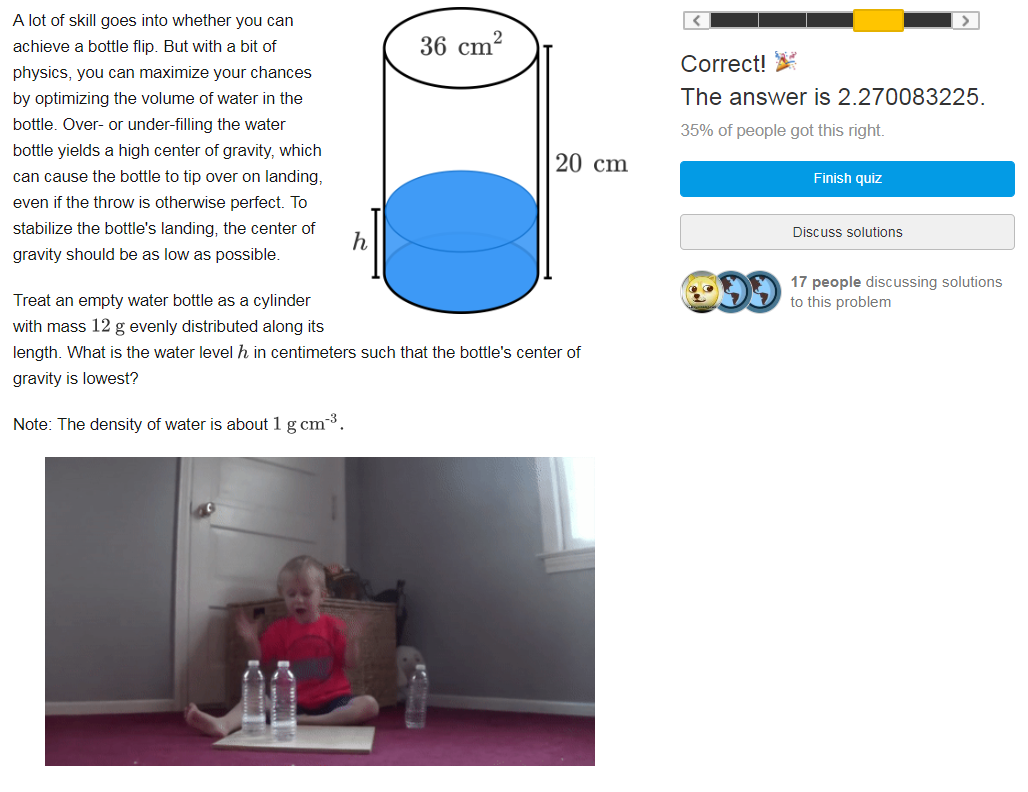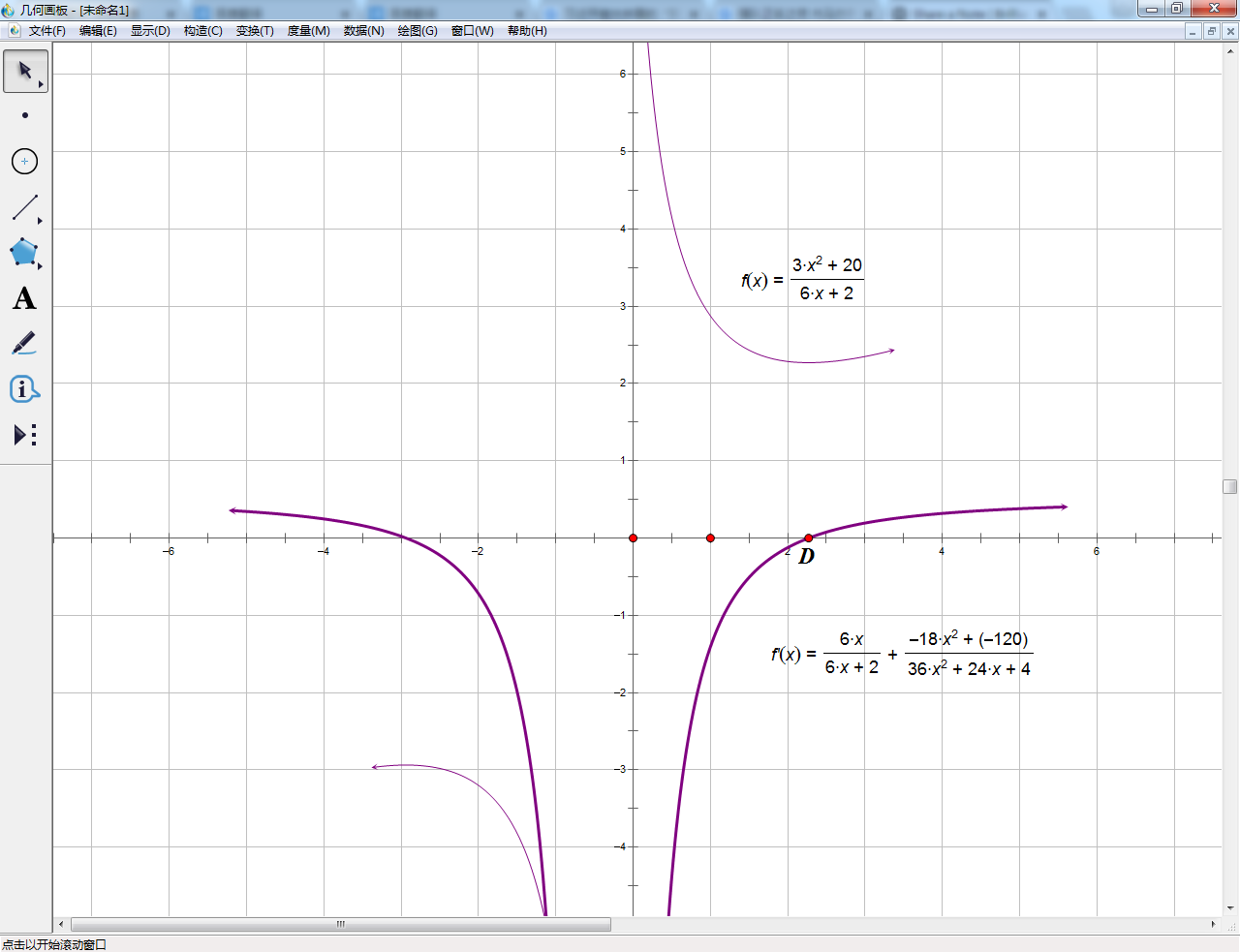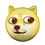# Use GSP to solve problems about FUNCTIONIn order to treat with this disgusting problem on the homepage,I have tried some different methods, including a few experiment. Finally I found a really EASY way. I first expressed the function of the height of the gravity with the parameter 'h'. f(h)=[36h(h/2)+120]/(36h+12)=3h^2+20/(6h+2) (h≥0) Then I expressed the derived function of f(h) (h≥0) and found out the points when the derived function is ZERO, while f(h) is at the smallest value.GSP is a very interesting and professional softwere which can build geometrical graphs, even the image of a function. So I can find the position of the "SMALLEST-VALUE-POINT" quickly by searching on the visible interface.I got the answer of 2.27.Though it cannot be completely precision, It's enough for this exercise and most other applications.Note by Yumi Zhang
3 years ago

This discussion board is a place to discuss our Daily Challenges and the math and science related to those challenges. Explanations are more than just a solution — they should explain the steps and thinking strategies that you used to obtain the solution. Comments should further the discussion of math and science.

When posting on Brilliant:

• Use the emojis to react to an explanation, whether you're congratulating a job well done , or just really confused .
• Ask specific questions about the challenge or the steps in somebody's explanation. Well-posed questions can add a lot to the discussion, but posting "I don't understand!" doesn't help anyone.
• Try to contribute something new to the discussion, whether it is an extension, generalization or other idea related to the challenge.

MarkdownAppears as
*italics* or _italics_ italics
**bold** or __bold__ bold
- bulleted- list
• bulleted
• list
1. numbered2. list
1. numbered
2. list
Note: you must add a full line of space before and after lists for them to show up correctly
paragraph 1paragraph 2

paragraph 1

paragraph 2

[example link](https://brilliant.org)example link
> This is a quote
This is a quote
    # I indented these lines
# 4 spaces, and now they show
# up as a code block.

print "hello world"
# I indented these lines
# 4 spaces, and now they show
# up as a code block.

print "hello world"
MathAppears as
Remember to wrap math in $$ ... $$ or $ ... $ to ensure proper formatting.
2 \times 3 $2 \times 3$
2^{34} $2^{34}$
a_{i-1} $a_{i-1}$
\frac{2}{3} $\frac{2}{3}$
\sqrt{2} $\sqrt{2}$
\sum_{i=1}^3 $\sum_{i=1}^3$
\sin \theta $\sin \theta$
\boxed{123} $\boxed{123}$

Sort by:

- 3 years ago